HomeUncategorizedFake news detection with and without code using data science

# Fake news detection with and without code using data science

 Audio version of the article

# Solving the problem with Python

```fake = pd.read_csv("data/Fake.csv")
```fake['target'] = 'fake'
true['target'] = 'true'```
`data = pd.concat([fake, true]).reset_index(drop = True)`
```from sklearn.utils import shuffle
data = shuffle(data)
data = data.reset_index(drop=True)```

## Data cleansing

`data.drop(["date"],axis=1,inplace=True)`
`data.drop(["title"],axis=1,inplace=True)`
`data['text'] = data['text'].apply(lambda x: x.lower())`
```import stringdef punctuation_removal(text):
all_list = [char for char in text if char not in string.punctuation]
clean_str = ''.join(all_list)
return clean_strdata['text'] = data['text'].apply(punctuation_removal)```
```import nltk
from nltk.corpus import stopwords
stop = stopwords.words('english')data['text'] = data['text'].apply(lambda x: ' '.join([word for word in x.split() if word not in (stop)]))```

## Data Exploration

```print(data.groupby(['subject'])['text'].count())
data.groupby(['subject'])['text'].count().plot(kind="bar")
plt.show()```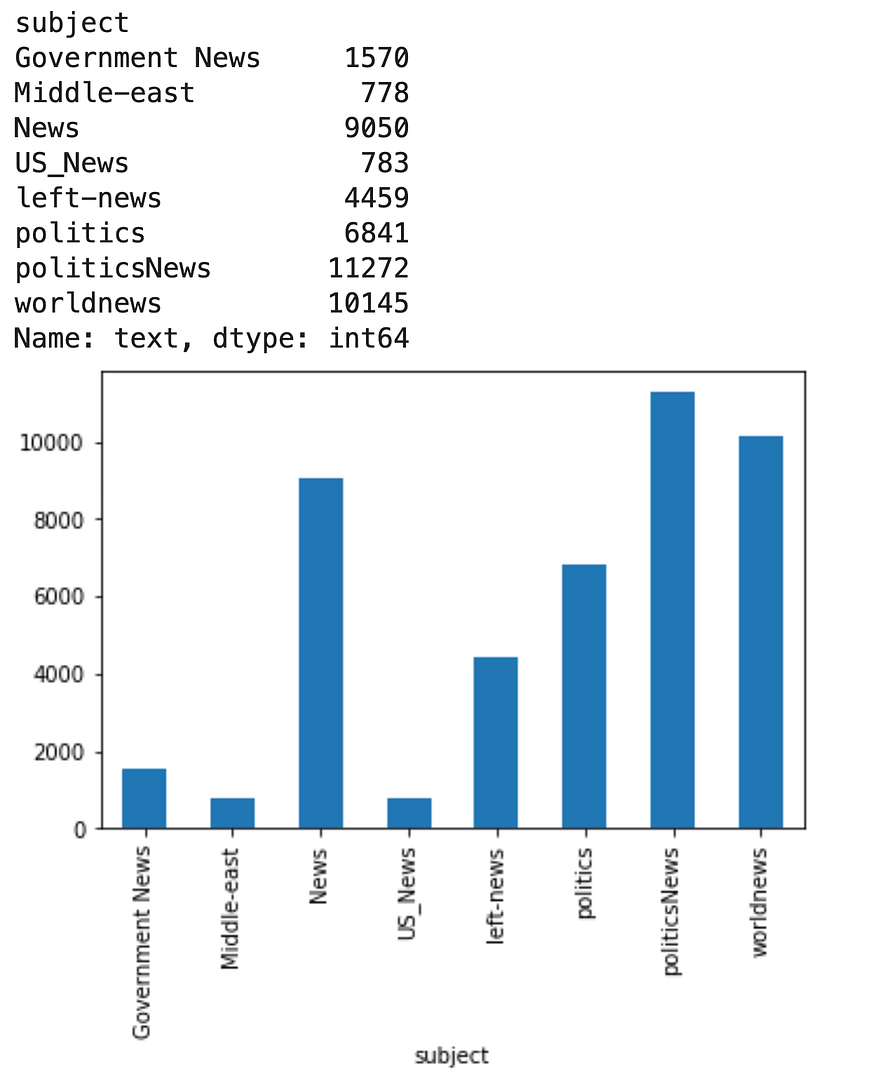```print(data.groupby([‘target’])[‘text’].count())
data.groupby([‘target’])[‘text’].count().plot(kind=”bar”)
plt.show()```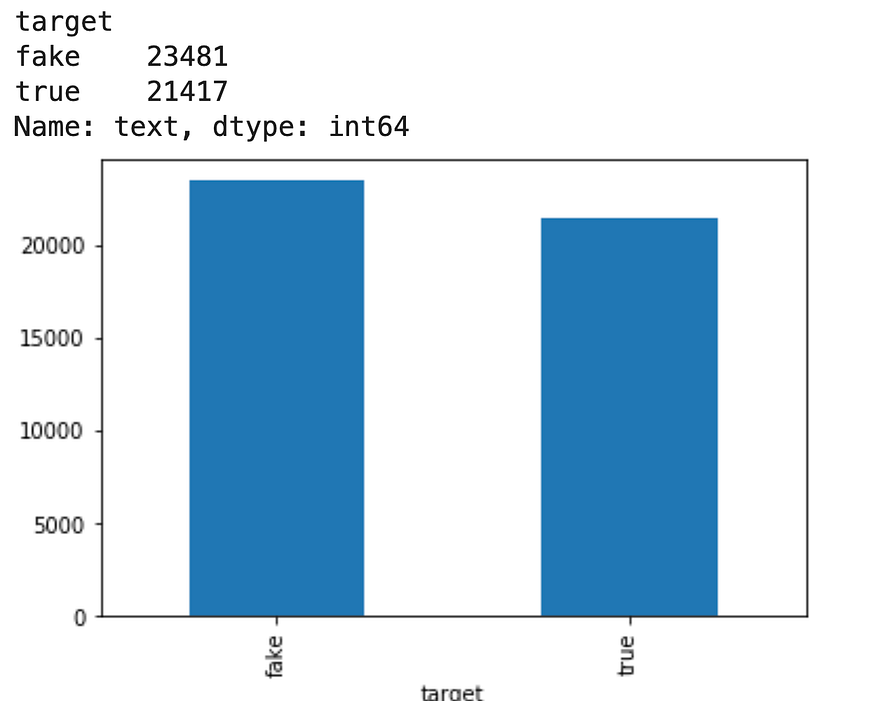```from wordcloud import WordCloudfake_data = data[data["target"] == "fake"]
all_words = ' '.join([text for text in fake_data.text])wordcloud = WordCloud(width= 800, height= 500,
max_font_size = 110,
collocations = False).generate(all_words)plt.figure(figsize=(10,7))
plt.imshow(wordcloud, interpolation='bilinear')
plt.axis("off")
plt.show()```
```from wordcloud import WordCloudreal_data = data[data[“target”] == “true”]
all_words = ‘ ‘.join([text for text in fake_data.text])wordcloud = WordCloud(width= 800, height= 500, max_font_size = 110,
collocations = False).generate(all_words)plt.figure(figsize=(10,7))
plt.imshow(wordcloud, interpolation=’bilinear’)
plt.axis(“off”)
plt.show()```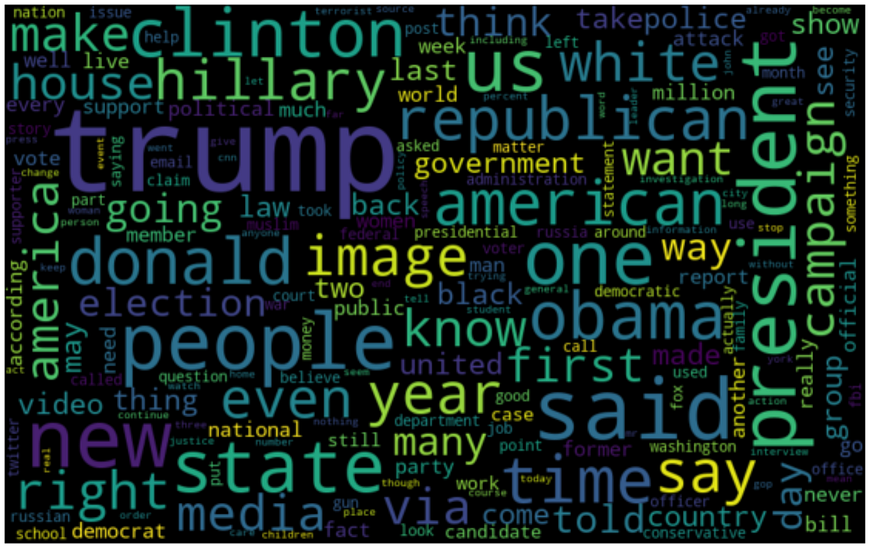```# Most frequent words counter (Code adapted from https://www.kaggle.com/rodolfoluna/fake-news-detector)
from nltk import tokenizetoken_space = tokenize.WhitespaceTokenizer()def counter(text, column_text, quantity):
all_words = ' '.join([text for text in text[column_text]])
token_phrase = token_space.tokenize(all_words)
frequency = nltk.FreqDist(token_phrase)
df_frequency = pd.DataFrame({"Word": list(frequency.keys()),
"Frequency": list(frequency.values())})
df_frequency = df_frequency.nlargest(columns = "Frequency", n = quantity)
plt.figure(figsize=(12,8))
ax = sns.barplot(data = df_frequency, x = "Word", y = "Frequency", color = 'blue')
ax.set(ylabel = "Count")
plt.xticks(rotation='vertical')
plt.show()```
`counter(data[data[“target”] == “fake”], “text”, 20)`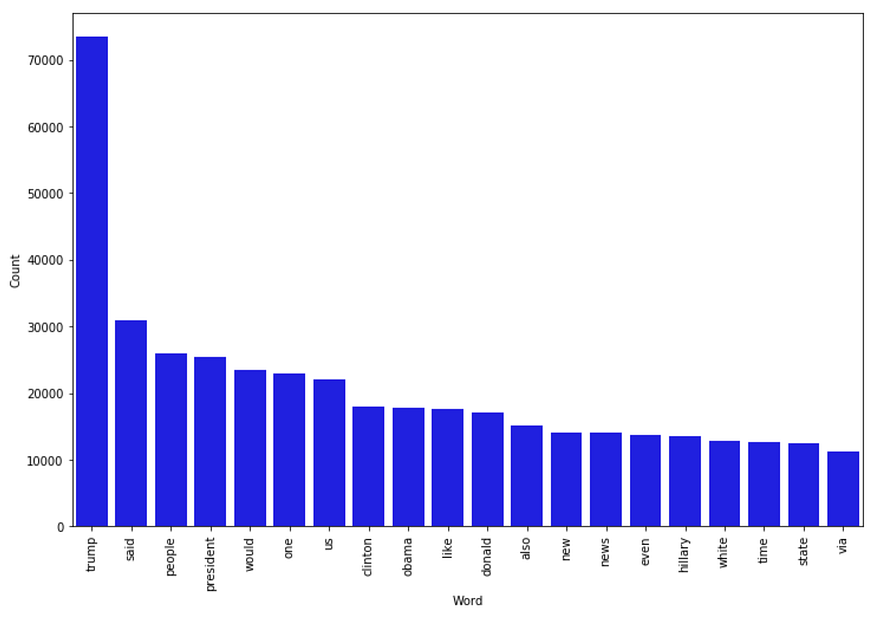`counter(data[data[“target”] == “true”], “text”, 20)`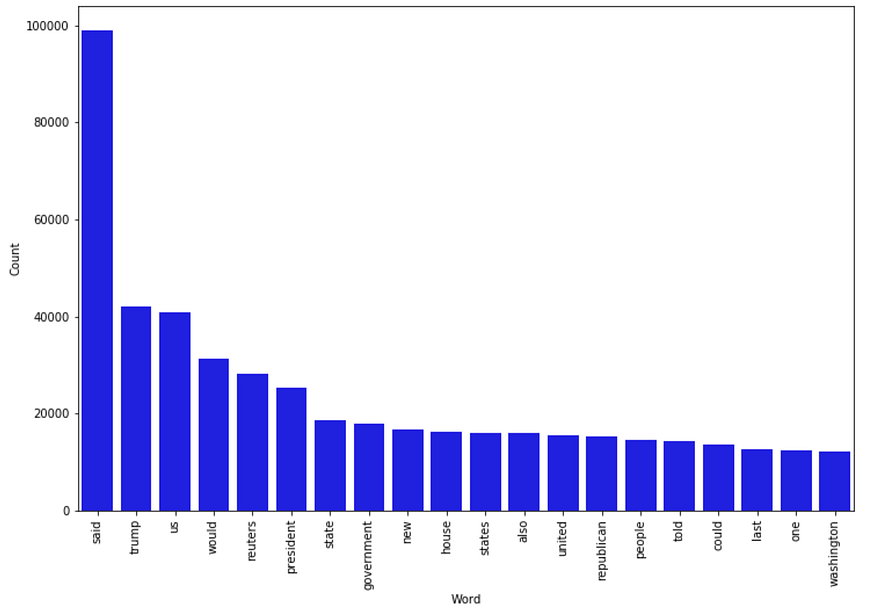## Modeling

```# Function to plot the confusion matrix (code from https://scikit-learn.org/stable/auto_examples/model_selection/plot_confusion_matrix.html)
from sklearn import metrics
import itertoolsdef plot_confusion_matrix(cm, classes,
normalize=False,
title='Confusion matrix',
cmap=plt.cm.Blues):

plt.imshow(cm, interpolation='nearest', cmap=cmap)
plt.title(title)
plt.colorbar()
tick_marks = np.arange(len(classes))
plt.xticks(tick_marks, classes, rotation=45)
plt.yticks(tick_marks, classes)if normalize:
cm = cm.astype('float') / cm.sum(axis=1)[:, np.newaxis]
print("Normalized confusion matrix")
else:
print('Confusion matrix, without normalization')thresh = cm.max() / 2.
for i, j in itertools.product(range(cm.shape), range(cm.shape)):
plt.text(j, i, cm[i, j],
horizontalalignment="center",
color="white" if cm[i, j] > thresh else "black")plt.tight_layout()
plt.ylabel('True label')
plt.xlabel('Predicted label')```
`X_train,X_test,y_train,y_test = train_test_split(data['text'], data.target, test_size=0.2, random_state=42)`
```# Vectorizing and applying TF-IDF
from sklearn.linear_model import LogisticRegressionpipe = Pipeline([('vect', CountVectorizer()),
('tfidf', TfidfTransformer()),
('model', LogisticRegression())])# Fitting the model
model = pipe.fit(X_train, y_train)# Accuracy
prediction = model.predict(X_test)
print("accuracy: {}%".format(round(accuracy_score(y_test, prediction)*100,2)))```
```cm = metrics.confusion_matrix(y_test, prediction)
plot_confusion_matrix(cm, classes=['Fake', 'Real'])```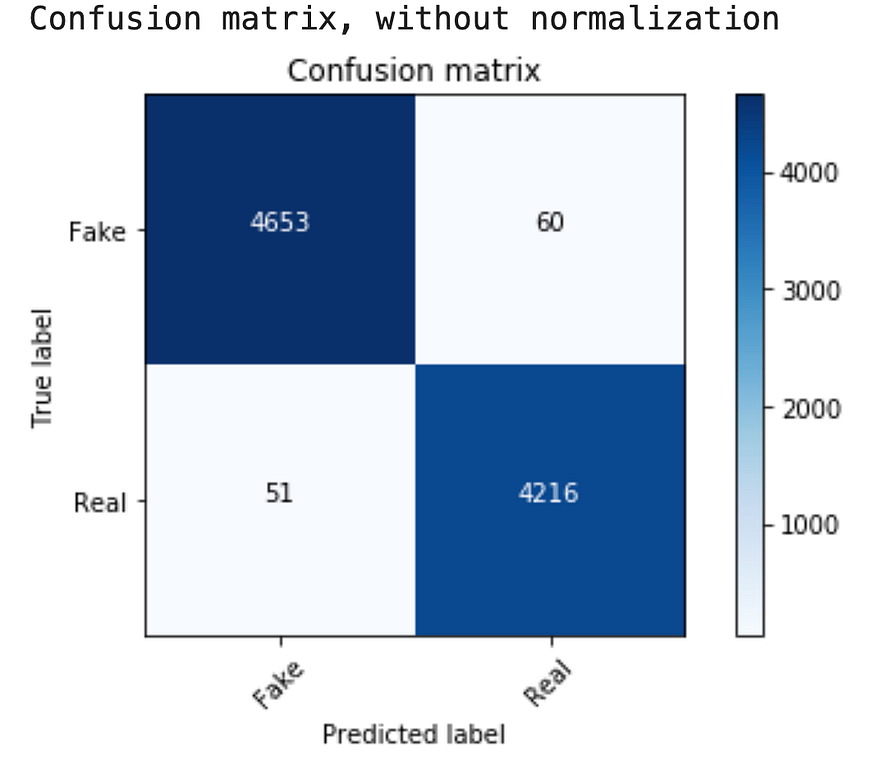```from sklearn.tree import DecisionTreeClassifier# Vectorizing and applying TF-IDF
pipe = Pipeline([('vect', CountVectorizer()),
('tfidf', TfidfTransformer()),
('model', DecisionTreeClassifier(criterion= 'entropy',
max_depth = 20,
splitter='best',
random_state=42))])
# Fitting the model
model = pipe.fit(X_train, y_train)# Accuracy
prediction = model.predict(X_test)
print("accuracy: {}%".format(round(accuracy_score(y_test, prediction)*100,2)))```
```cm = metrics.confusion_matrix(y_test, prediction)
plot_confusion_matrix(cm, classes=['Fake', 'Real'])```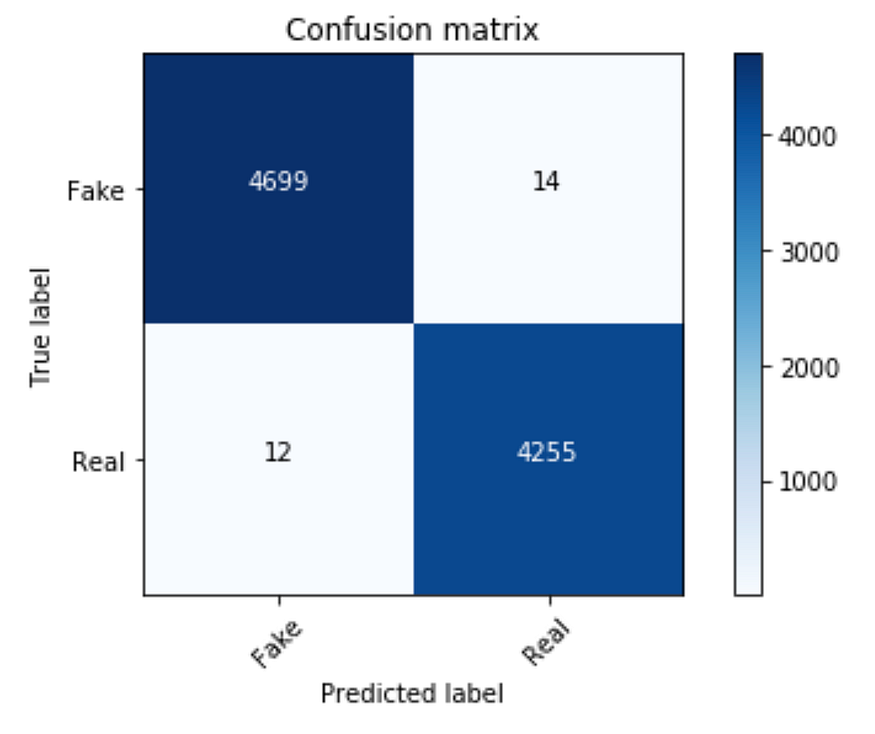```from sklearn.ensemble import RandomForestClassifierpipe = Pipeline([('vect', CountVectorizer()),
('tfidf', TfidfTransformer()),
('model', RandomForestClassifier(n_estimators=50, criterion="entropy"))])model = pipe.fit(X_train, y_train)
prediction = model.predict(X_test)
print("accuracy: {}%".format(round(accuracy_score(y_test, prediction)*100,2)))```
```cm = metrics.confusion_matrix(y_test, prediction)
plot_confusion_matrix(cm, classes=['Fake', 'Real'])```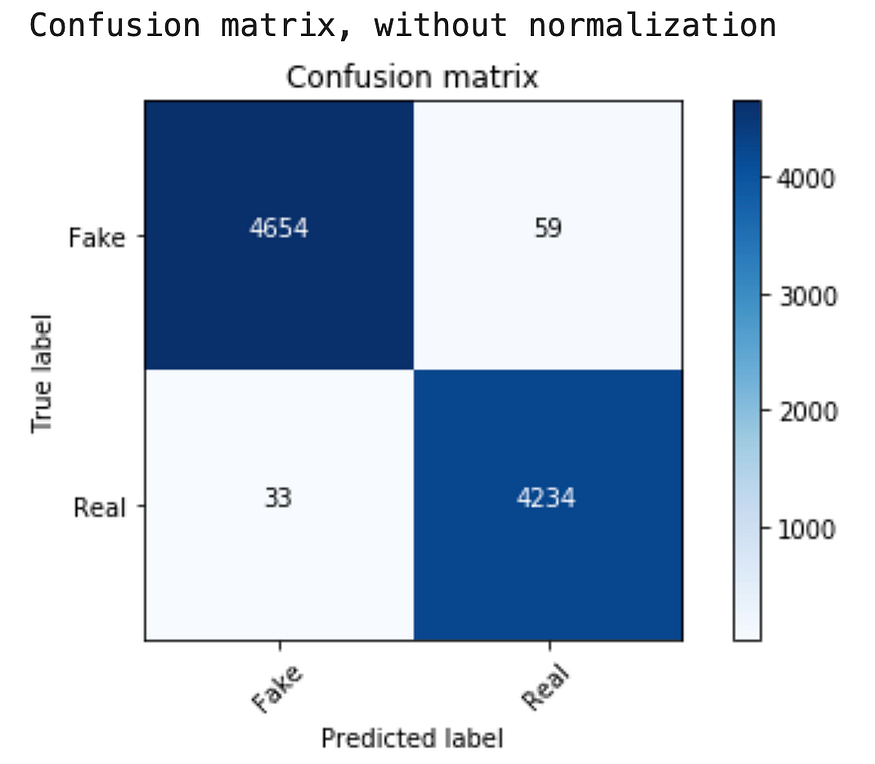# Solving the problem without coding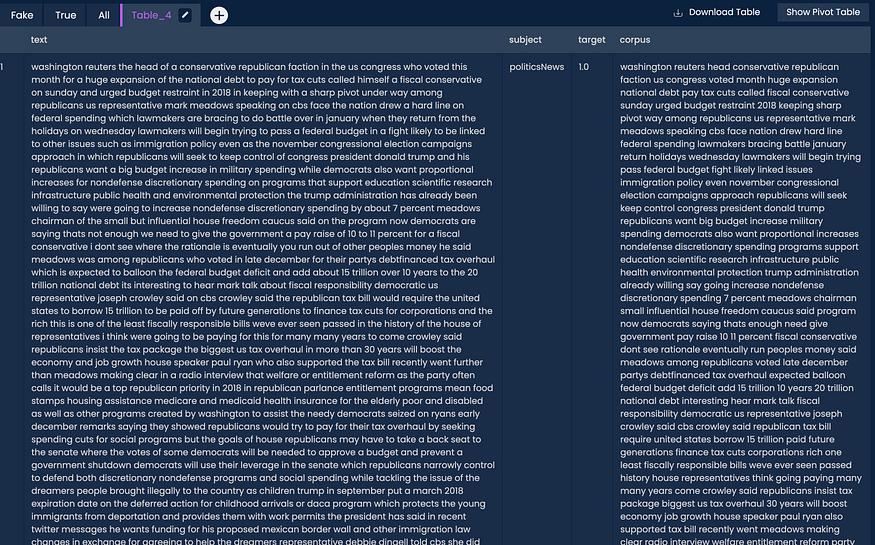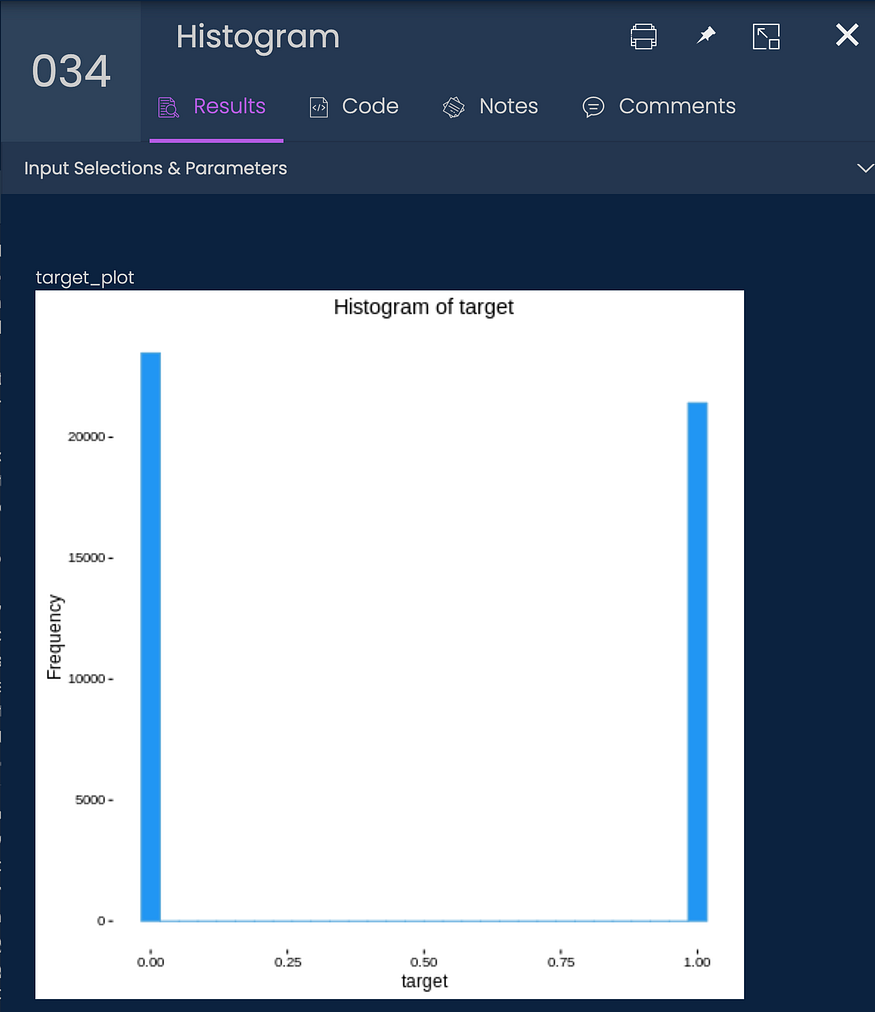# Conclusions

- Advertisment -### A Brief Introduction To Blockchain Merkle Tree

- Advertisment -